# Surds and Indices FormulasIndices: - When a number (let a) is multiplied by itself “n” times. For example an
Here ‘a’ is called the base and ‘n’ is known as the index of the power. Basically it is an exponential expression.

## Rules of Indices: -Indices: - Any root of a non negative rational number, which can not be found or does not provide an exact solution. For example n√a
Here ‘a’ is called as radicand and ‘n’ is known as the order of surds. ‘n’ should be a natural number.
Note: - All surds are irrational number but all irrational number are not surds.

### Order of the surds: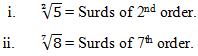Note: -
a) Surds of 2nd order are known as quadratic surds.
b) Surds of 3rd order are known as cubic surds.

## Rules of Surds: -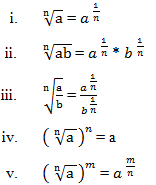### Type of Surds: -

• Pure Surds:-  Those surds which do not have factor other than 1. For example 2√3, 3√7
• Mixed Surds:- Those surds which do not have factor than 1. For example √27 = 3√3, √50 = 5√2
• Similar Surds:- When the radicands of two surds are same. For example 5√2 and 7√2
• Unlike Surds:- When the radicands are different. For example √2 and 2√5

### Formulas/ Patterns Including Short Tricks:

#### 1) Arrangement of surds in either increasing or decreasing order –

First of all, take the LCM of the denominator of the powers and use it to make them same and then solve it.
Example: -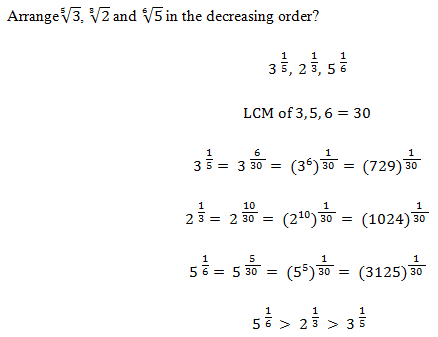#### 2) Calculation on indices/ surds to find the value of any expression –

In such questions, factorize the expression in the smallest possible number then solve the expression using the rules of surds/ indices.
Example: -#### 3) Addition operation on surds –In such questions, break the x into m (m + 1) form then answer would be (m + 1).
Example: -#### 4) Subtraction operation on surds –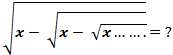In such questions, factorize the x into m (m + 1) form then ‘m’ would be the answer.
Example: -#### 5) Multiplication operation on surds –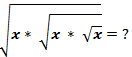In such questions, answer would beWhere n = number of time x is repeated.
Example: -#### 6) Rationalization on surds –

In this process, we convert the denominator of the surds into a rational number. For this we have to multiply both numerator and denominator of the surds with another surd and obtain rational number by applying formula (a + b) * (a – b) = (a2- b2)Example: -
Find the value of#### What's trending in BankExamsToday

Smart Prep Kit for Banking Exams by Ramandeep Singh - Download here